# High School Math : Simplifying Equations

## Example Questions

### Example Question #1 : Simplifying Equations

Simplify the fraction to the lowest terms: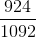Cannot be simplified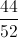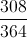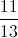Explanation:

Find the common multiple between the numerator and denominator.divide numerator and denominator by 3: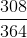divide numerator and denominator by 7: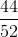divide numerator and denominator by 4: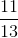Cannot be divided any more- lowest terms.

### Example Question #2 : Simplifying Equations

Solve the following equation for x in terms of the other variables: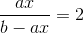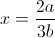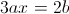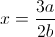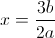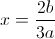Explanation:Multiply both sides by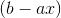to get: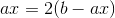Distribute the: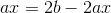Combine like terms:Divide both sides by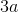: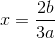### Example Question #3 : Simplifying Equations

Solve the following equation for x in terms of the other variables: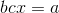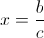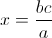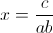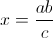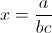Explanation:Divide both sides by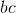: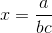### Example Question #1 : Equations

If given the equation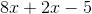, witha positive integer, the result must be an integer multiple of:

8

10

2

5

12

The mathematical expression given in the question is. Adding together like terms,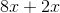, this can be simplified to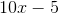. The expressioncan be factored as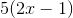. For every positive integer,must be a multiple of 5. If, then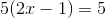, which is not an integer multiple of 2, 8, 10, or 15. Therefore, the correct answer is 5.